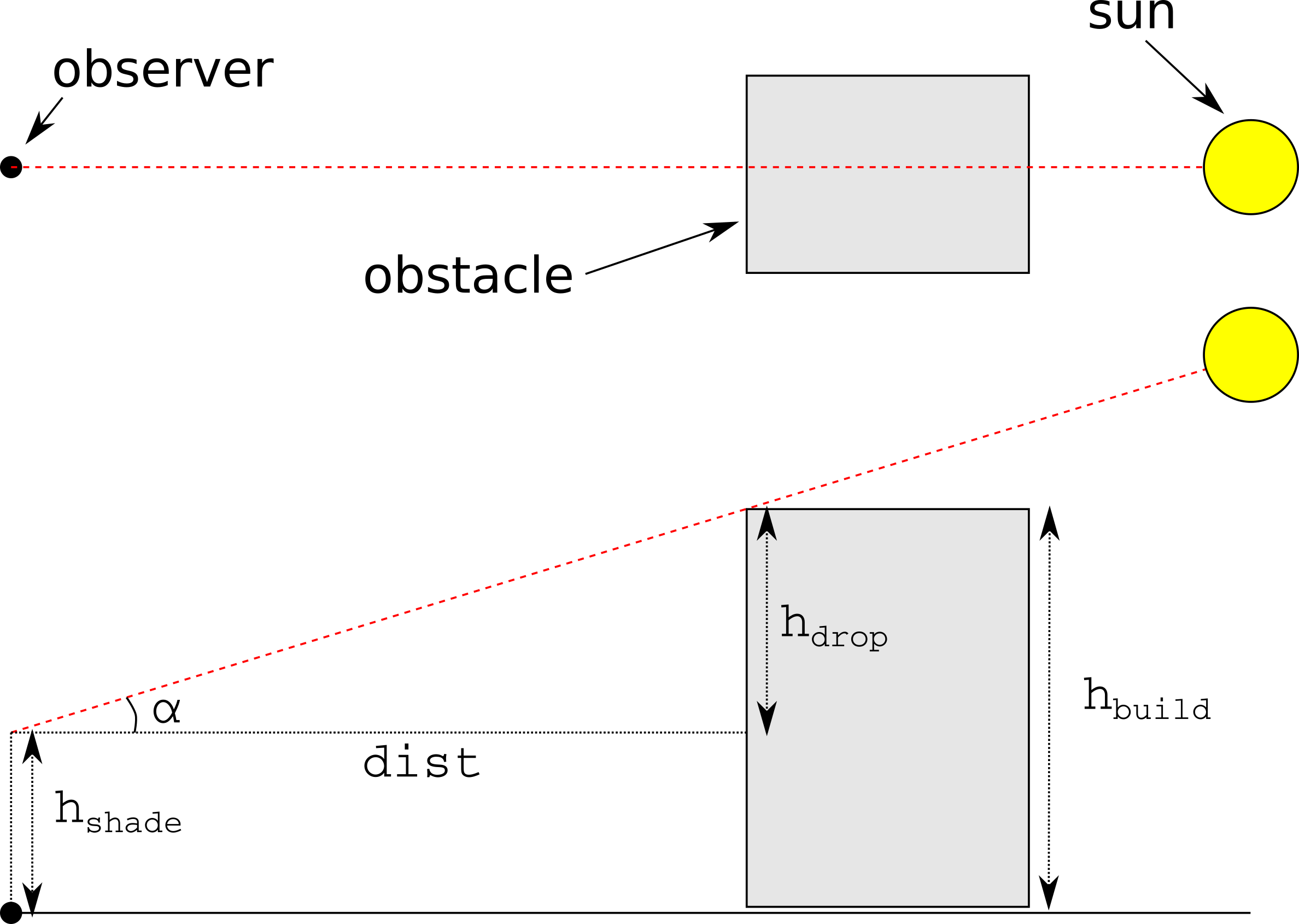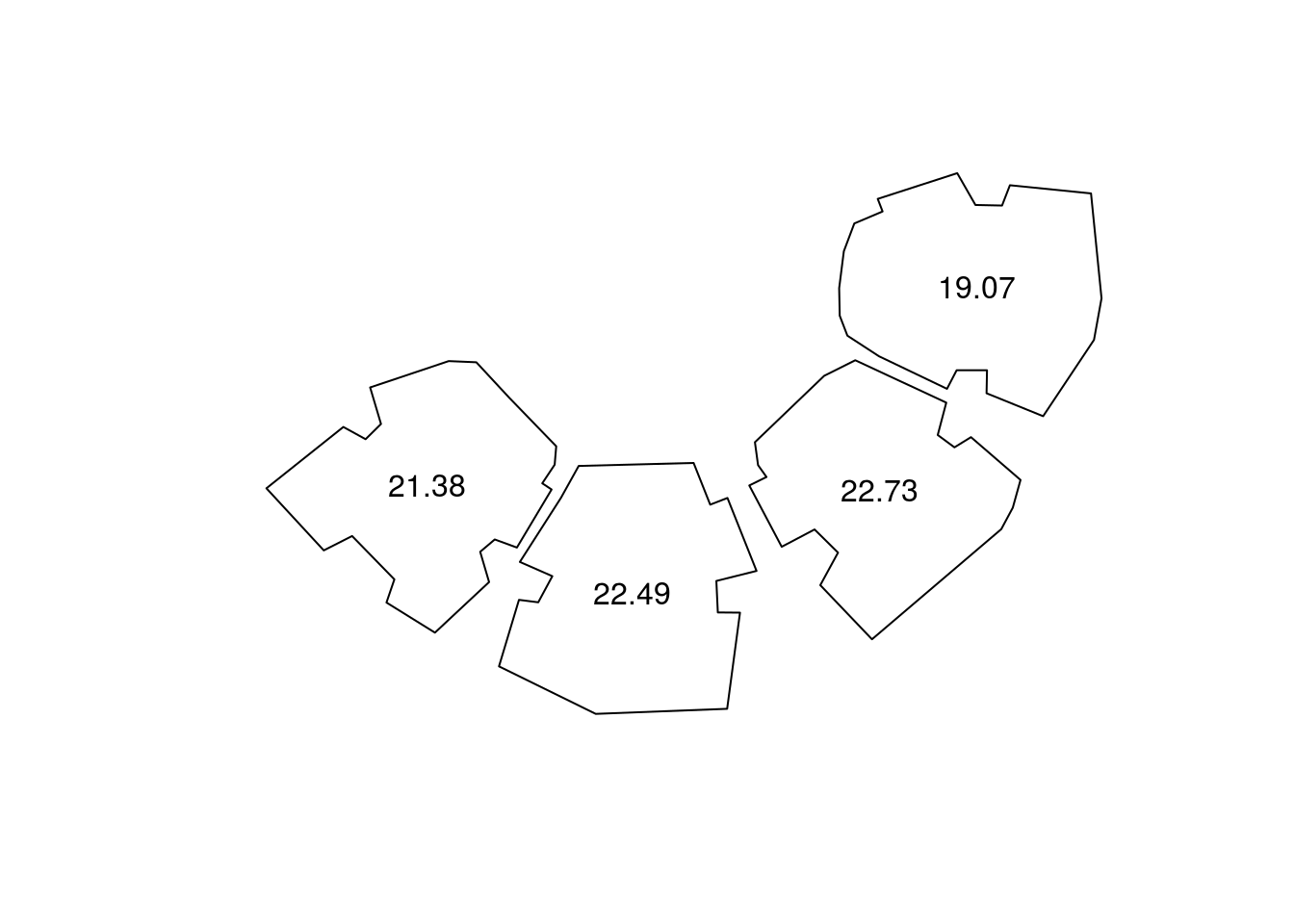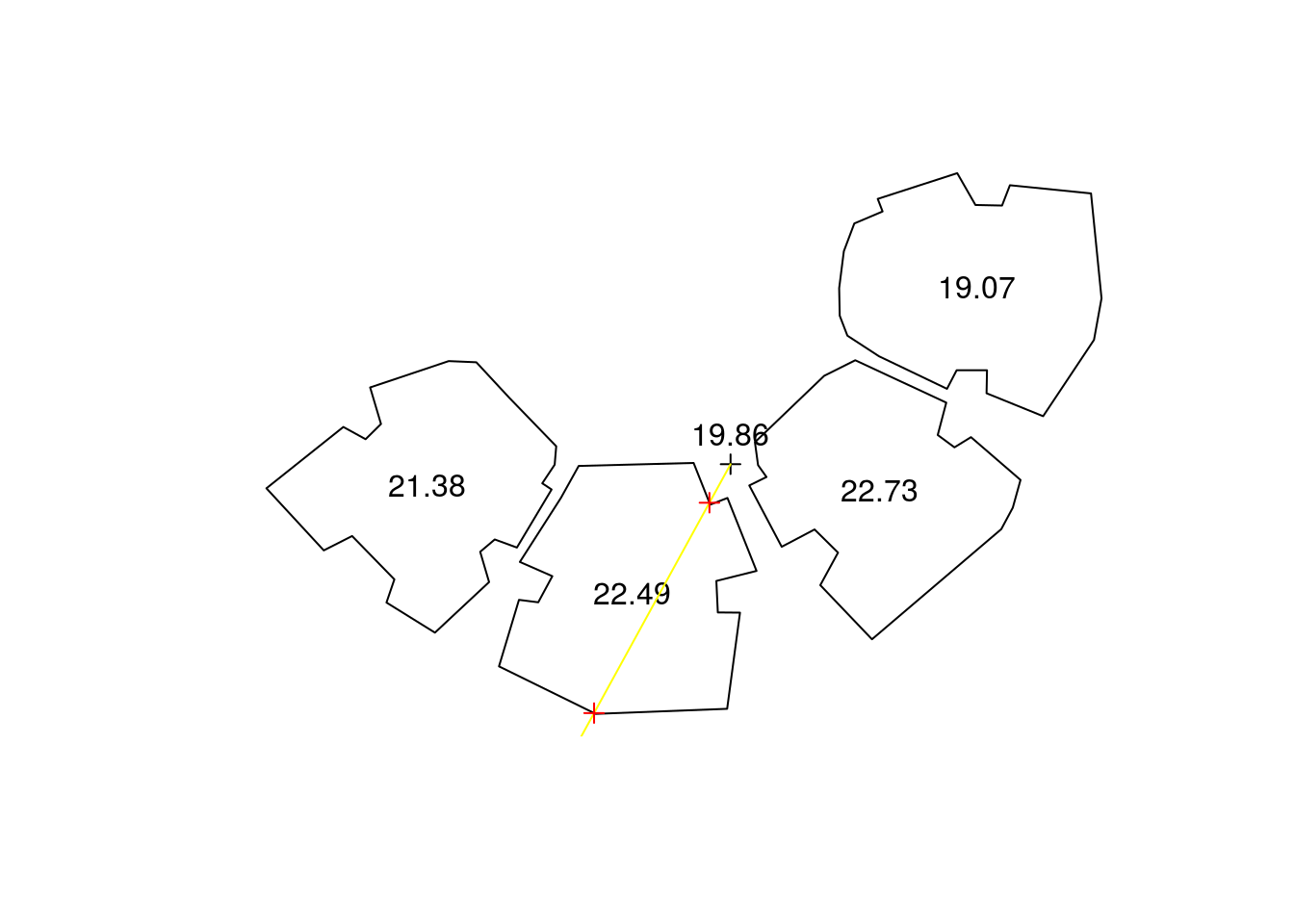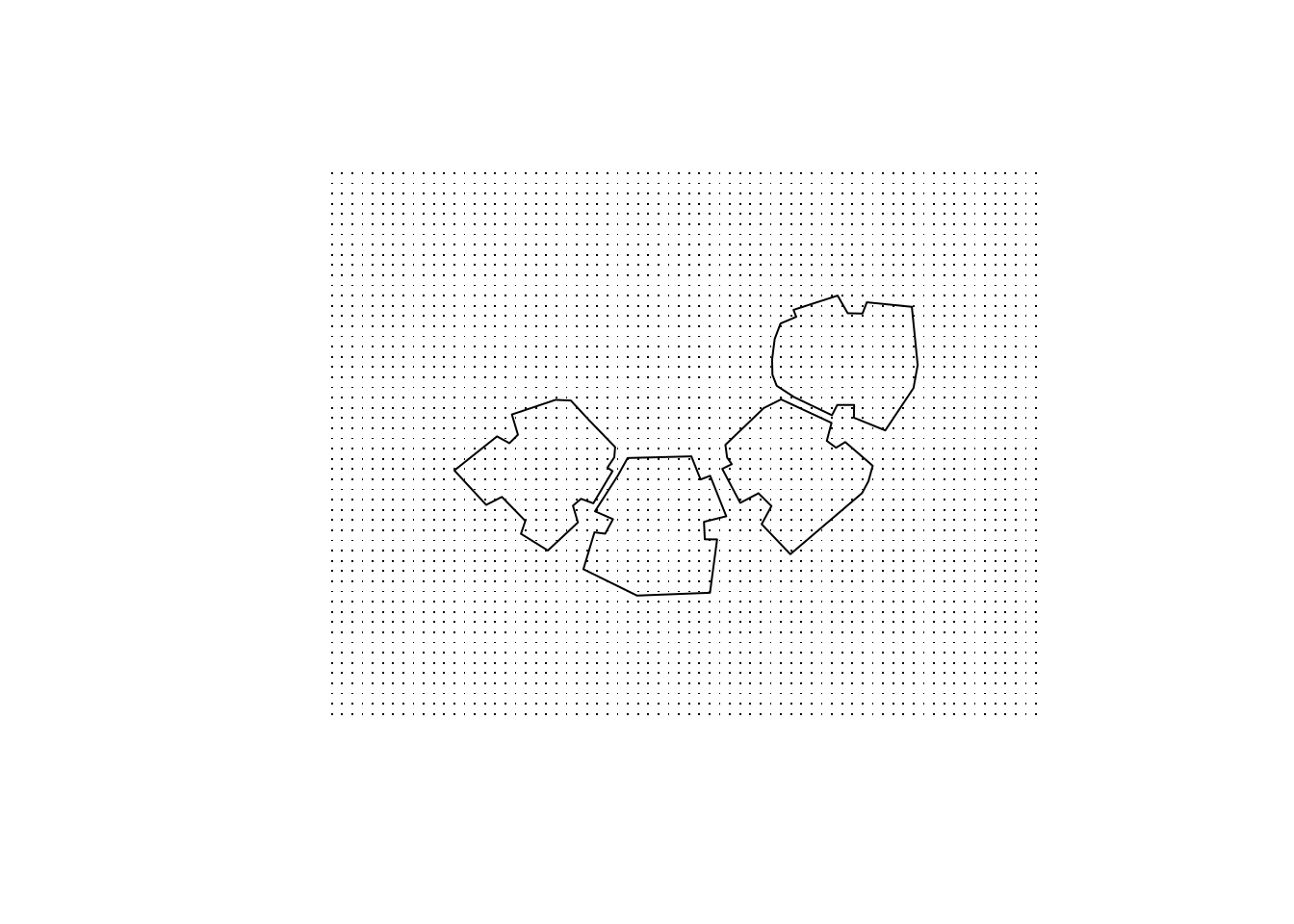# 1 Introduction

Spatial analysis of the urban environment frequently requires estimating shading. For example -

• The amount of time a given point / roof / facade is shaded may determine the utility of installing Photo-Voltaic cells for electricity production

• The Sky View Factor (SVF) of a given street is associated with its microclimate

• Calculating shade footprint on a green park helps urban planners provide guidelines for maximal height of a new building

These types of calculations (and more advanced ones) are usually restricted to proprietary software dealing with 3D models, such as ESRI’s ArcScene. Terrain-based solutions (i.e. Digital Elevation Model, DEM) are more common, in both open-source (GRASS GIS) as well as proprietary (ArcGIS) software. The insol R package (Corripio 2014) provides such capabilities in R. However terrain-based approaches may not be appropriate for an urban environment, for two reasons. First, a continuous elevation surface at the necessary resolution for the urban context (e.g. LIDAR) may not be available and is expensive to produce. Second, the DEMs cannot adequately represent individual urban elements such as building facades, thus limiting the interpretability of results. The shadow package aims at addressing these limitations. The shadow package operates on a vector layer of building outlines along with their heights, rather than a DEM. Such data are generally much more available, either from local municipalities or from global datasets such as OpenStreetMap. Therefore the resulting shade estimates correspond to urban environment such as individual buildings or facades. It should be noted that the approach assumes a flat terrain and no obstacles (e.g. trees) other than the buildings, which may be inappropriate in certain situations (e.g. a mountainous urban area).

## 1.1 Calculation

The functions currently included shadow are based on the trigonometric relations in the triangle defined by the sun rays, the ground (or plane parallel to the ground) and an obstacle. For example, as shown in Figure 1.1, shade height ($$h_{shade}$$) at a given location ($$observer$$) can be calculated based on the sun elevation angle ($$\alpha$$) in case there is one obstacle with a known height ($$h_{build}$$) and at a known distance ($$dist$$) -

$$h_{shade} = h_{build} - dist * tan(\alpha)$$

This approach can be expanded for the general case of multiple obstacles, in which case we must take the maximum value of all potential shade heights caused by the obstacles.# 2 Functions

The shadow package currently contains three main functions, as well as several helper functions. The main functions give three distinct aspects of shading -

• The shadeHeight function calculates shade height at a single point; the wrapper shadePropWall gives the shaded fraction of a facade, by repeatedly calling shadeHeight on sample points along its length.

• The shadeFootprint function calculates the shade footprint on the ground, given the layer of obstacles and sun position.

• The SVF function calculates the Sky View Factor (SVF), which is a measure of sky proportion not obstructed to the viewer at a given point on the ground.

# 3 Examples

Before going into the examples, we load the shadow package as well as packages sp (loaded automatically), raster and rgeos -

library(shadow)
library(raster)
library(rgeos)

In the examples we will use a polygonal layer representing four buildings (build) along having a height attribute (BLDG_HT) as shown on Figure 3.1 -

plot(build)
text(gCentroid(build, byid = TRUE), build$BLDG_HT)Figure 3.1: Sample buildings and heights ## 3.1 Shade height The shadeHeight function calculates shade height at a given point location. For example, to calculate shade height at the centroid of the layer (location), on 2004-12-24 13:30:00 we first need to determine the sun elevation and azimuth at that time. This can be done with function solarpos from package maptools - location = gCentroid(build) time = as.POSIXct("2004-12-24 13:30:00", tz = "Asia/Jerusalem") location_geo = spTransform(location, "+proj=longlat +datum=WGS84") solar_pos = maptools::solarpos(location_geo, time) solar_pos #> [,1] [,2] #> [1,] 208.7333 28.79944 Now we know the sun azimuth (208.7) and elevation (28.8). Given sun position, the layer of obstacles and queried location, shade height can be calculated with shadeHeight - h = shadeHeight(location, build, "BLDG_HT", solar_pos) #> Assuming BLDG_HT given in m h #>  19.86451 Shade height at the queried point is 19.86 meters. Note the warning regarding the units of the BLDG_HT attribute. The function has no way of knowing the height dimension units are the same as the Coordinate Reference System (CRS) spatial distance units. It is up to the user to make sure. The following code and subsequent Figure 3.2 illustrate how the calculation is carried out. First, a line of sight ray is drawn between the point of interest location and the sun position based on its azimuth sun_az. Potential intersections inter are then detected. Finally, the shade height induced by each intersection is calculated based on the distance to intersection, sun elevation sun_elev and building height. The final result is the maximum value of these potential heights. sun = shadow:::.sunLocation( location = location, sun_az = solar_pos[1, 1], sun_elev = solar_pos[1, 2] ) sun_ray = ray(from = location, to = sun) build_outline = as(build, "SpatialLinesDataFrame") inter = gIntersection(build_outline, sun_ray) plot(build) text(gCentroid(build, byid = TRUE), build$BLDG_HT)
text(
location,
pos = 3
)
plot(sun_ray, add = TRUE, col = "yellow")
plot(inter, add = TRUE, col = "red")Figure 3.2: Shade height at a single location

## 3.2 Calculating shade height for each point

The procedure can be readily expanded to calculate a continuous surface of shade heights. To make it faster, we can use mclapply from package parallel or any other parallelization solution.

First, we will create a grid covering the examined area with a spatial resolution of 2 meters -

ext = as(extent(build) + 50, "SpatialPolygons")
r = raster(ext, res = 2)
proj4string(r) = proj4string(build)
grid = rasterToPoints(r, spatial = TRUE)
grid = SpatialPointsDataFrame(
grid,
data.frame(grid_id = 1:length(grid))
)

The following code section plots the input grid (Figure 3.3).

plot(grid, pch = ".")
plot(build, add = TRUE)Figure 3.3: Input grid for creating a shade heights surface

Calculating shade height at each point, using package parallel -

library(parallel)
split(grid, grid$grid_id), shadeHeight, build, "BLDG_HT", solar_pos, mc.cores = 3 ) grid$shade_height = simplify2array(shade_heights)
The resulting grid can be converted to a RasterLayer object of shade heights and plotted with following code section (Figure 3.4). Note the partial shade on the roof of the 19.07-m building which is caused by the slightly taller 22.73-m building.
shade = as(grid, "SpatialPixelsDataFrame")
text(gCentroid(build, byid = TRUE), build\$BLDG_HT)